Constructing a copy of a given angle

Practical Geometry
Concept wise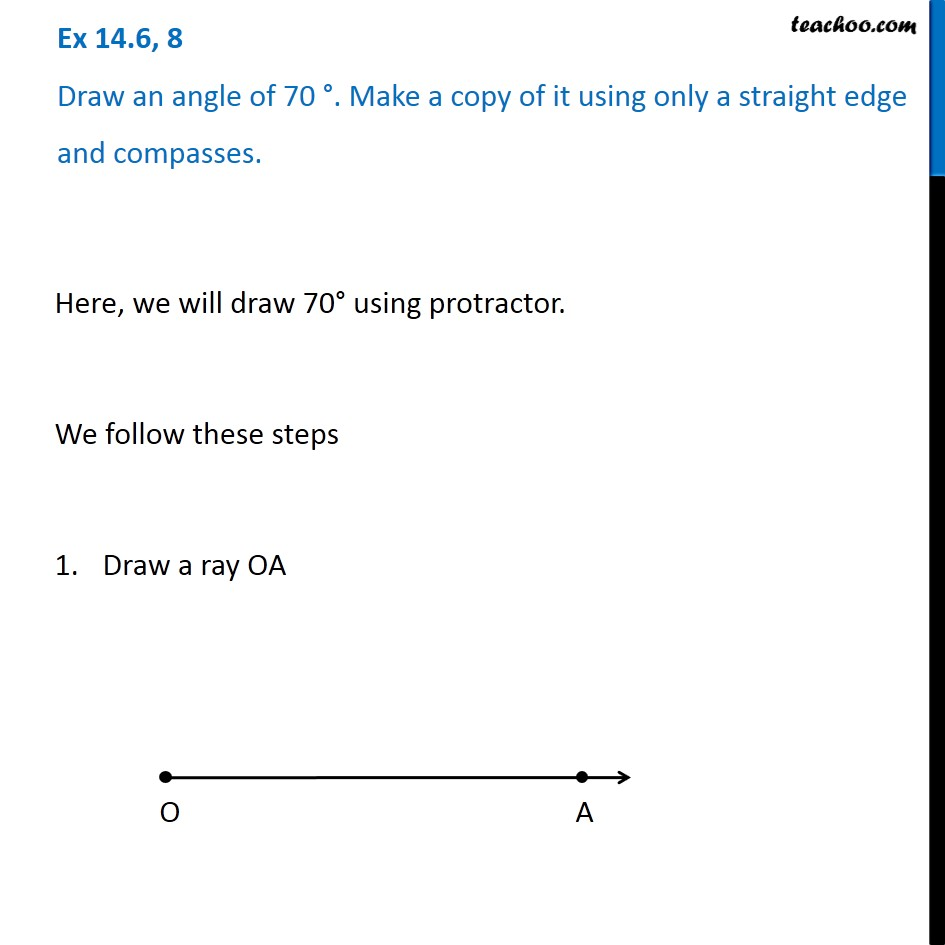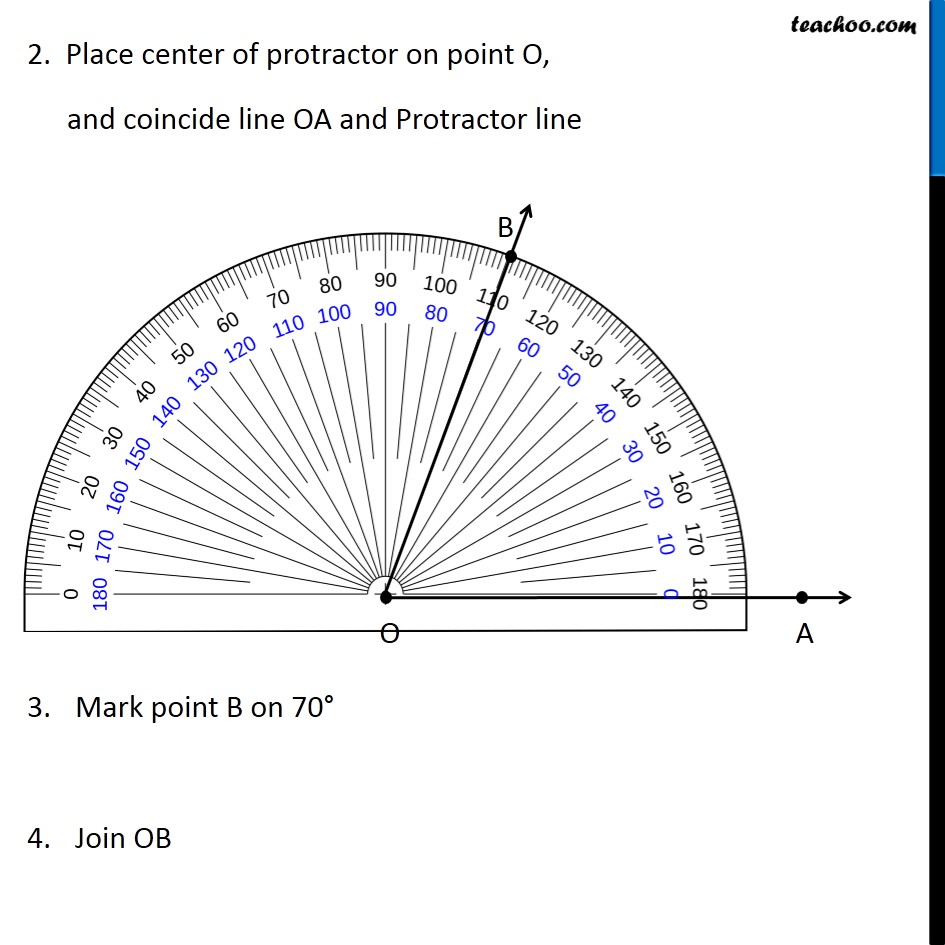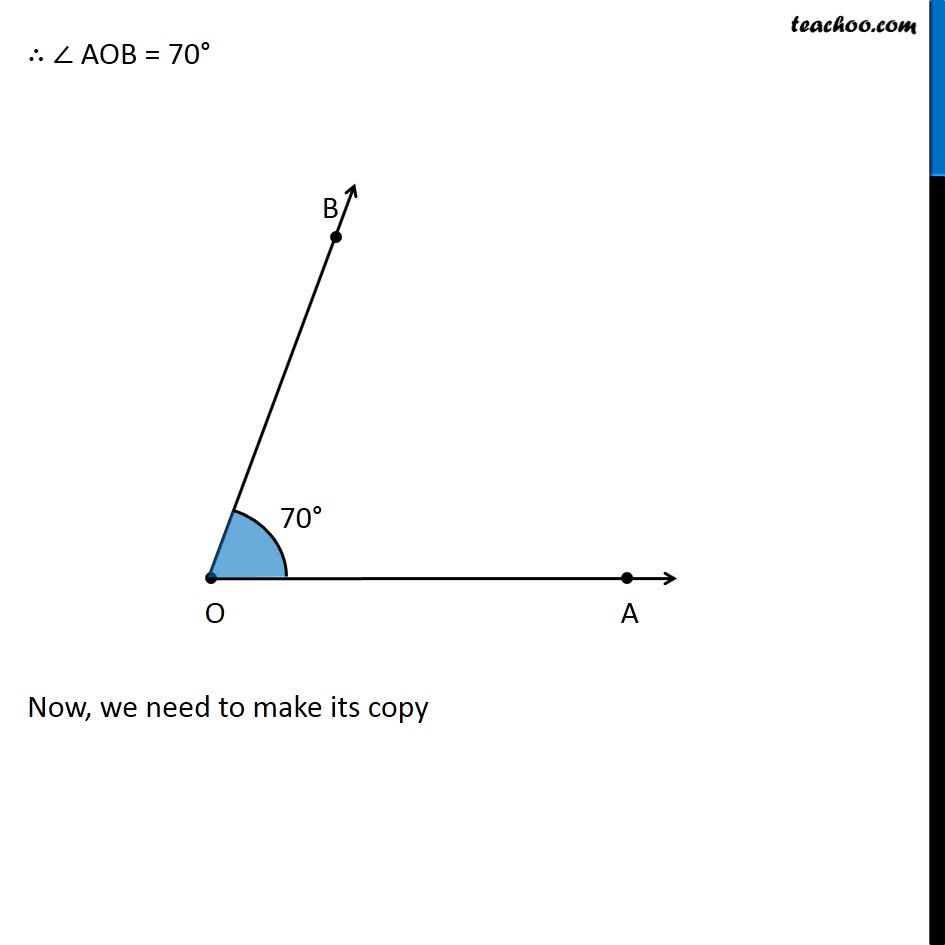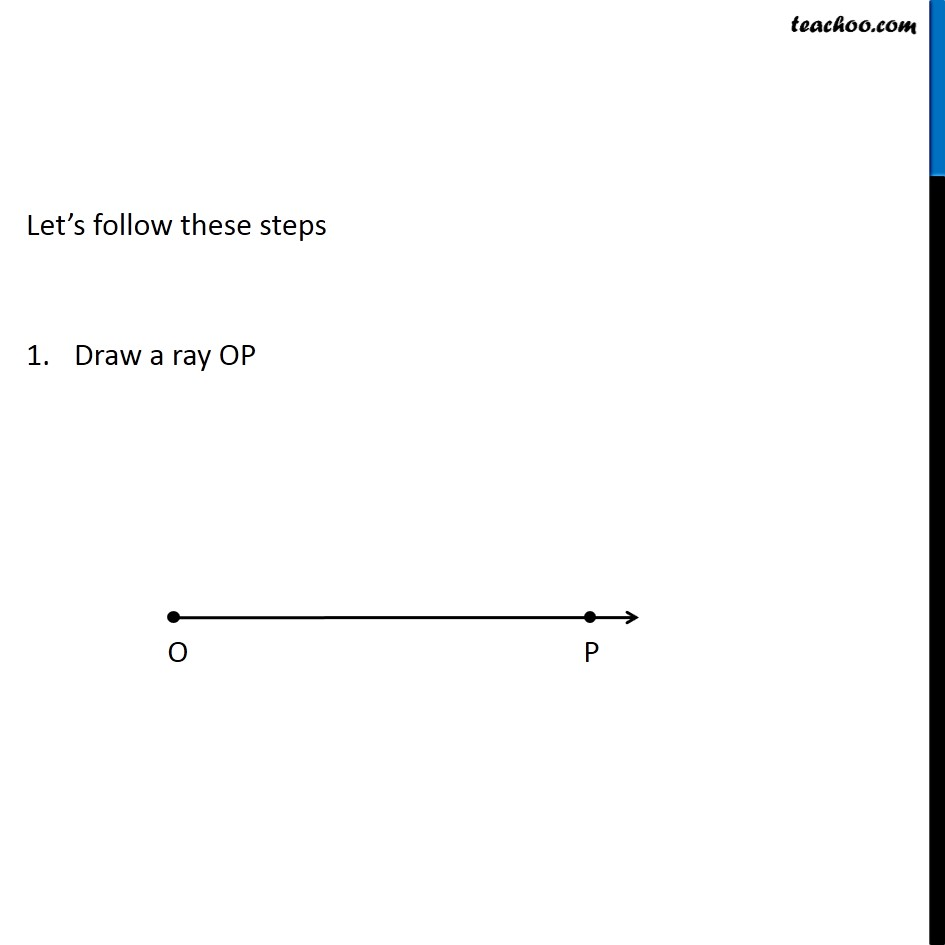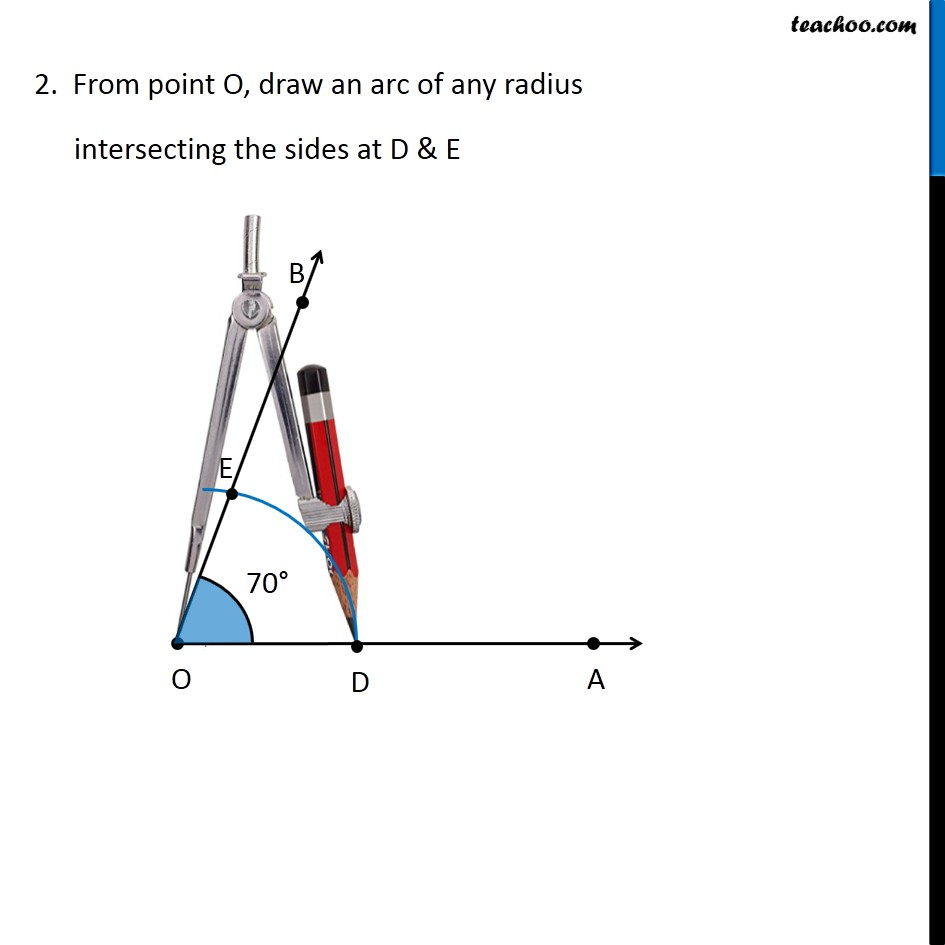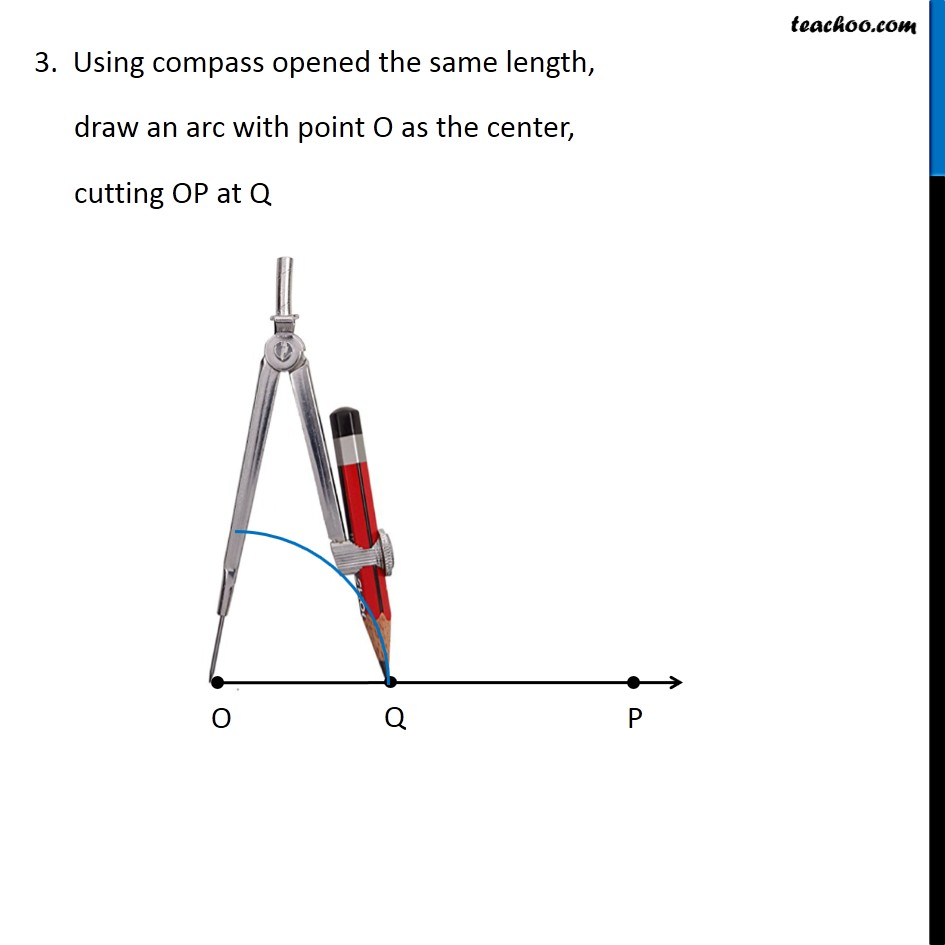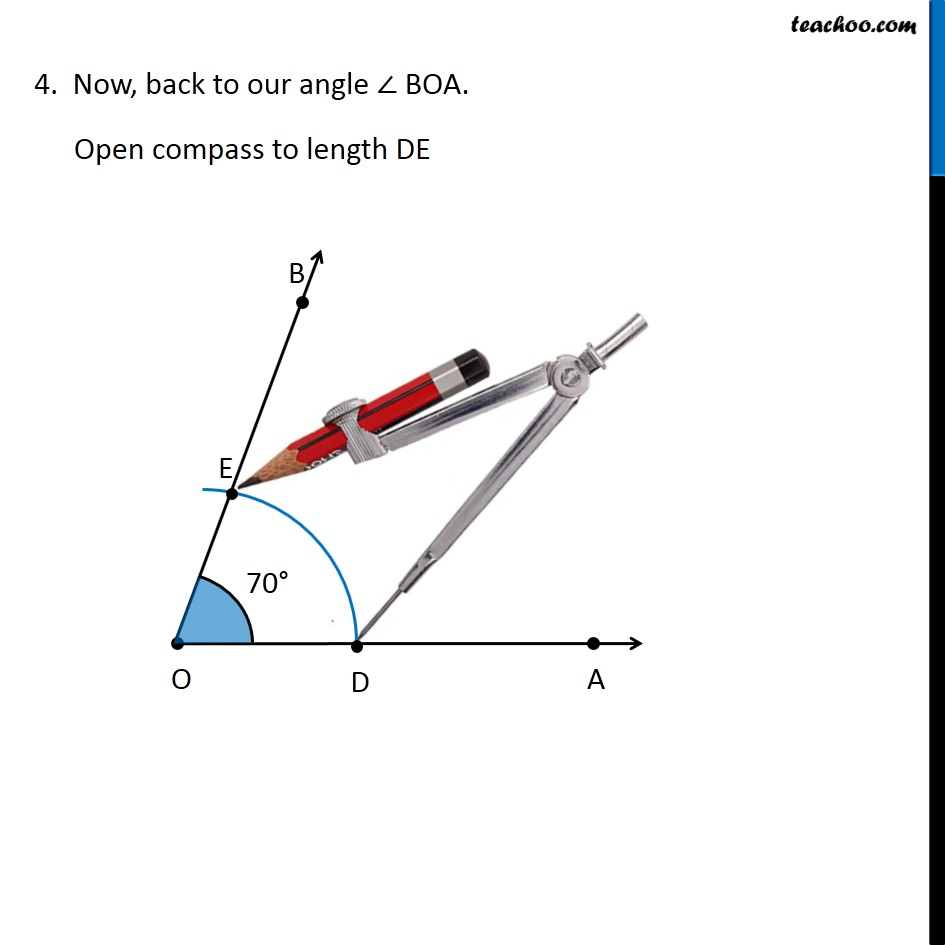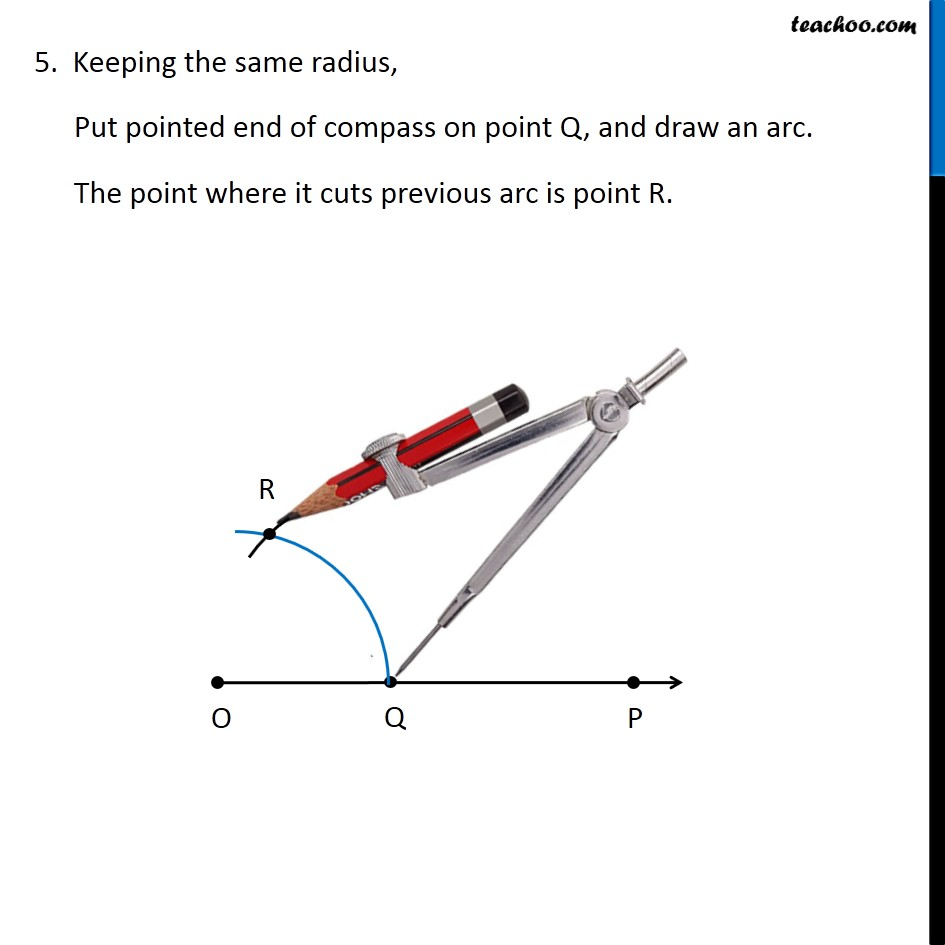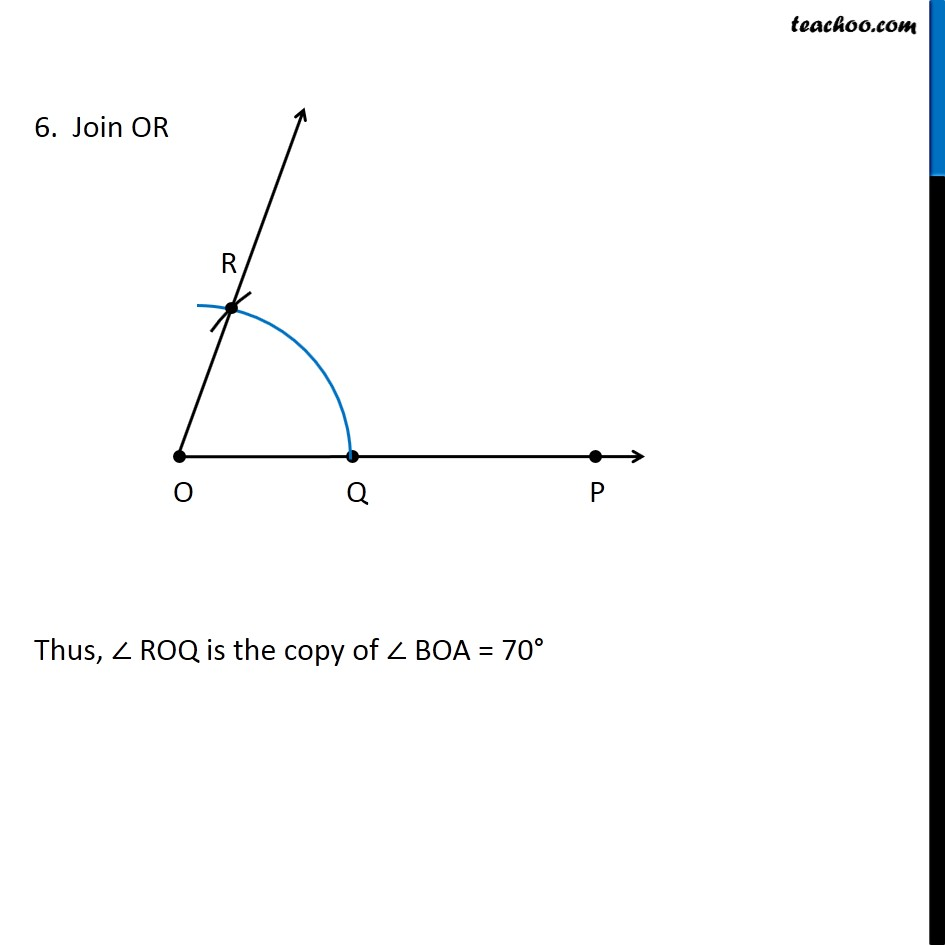Learn in your speed, with individual attention - Teachoo Maths 1-on-1 Class

### Transcript

Question 8 Draw an angle of 70 °. Make a copy of it using only a straight edge and compasses. Here, we will draw 70° using protractor. We follow these steps Draw a ray OA 2. Place center of protractor on point O, and coincide line OA and Protractor line Mark point B on 70° Join OB ∴ ∠ AOB = 70° Now, we need to make its copy Let’s follow these steps Draw a ray OP 2. From point O, draw an arc of any radius intersecting the sides at D & E 3. Using compass opened the same length, draw an arc with point O as the center, cutting OP at Q 4. Now, back to our angle ∠ BOA. Open compass to length DE 5. Keeping the same radius, Put pointed end of compass on point Q, and draw an arc. The point where it cuts previous arc is point R. 6. Join OR Thus, ∠ ROQ is the copy of ∠ BOA = 70°